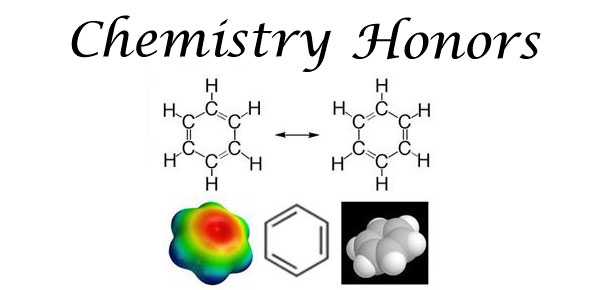# Honors Chemistry 2 Online Quiz 1

4 Questions | Total Attempts: 72SettingsGas Quiz. Honors Chemistry 2

Related Topics
• 1.
What are the conditions at STP?
• A.

1 atm and 25 degrees C

• B.

1 atm and 0 K

• C.

101.3 kPa and 0 degrees C

• D.

101.3 kPa and 25 K

• 2.
How many mmHg are equal to 25 torr?
• A.

760

• B.

1

• C.

25

• D.

73

• 3.
What is the formula for the combined gas law?
• A.

P1=((P2V2T1)/(T2V1)

• B.

P1V1=((P2V2)/(T2T1))

• C.

V1=((P2V2)/(T2T1P1)

• D.

PV=nRT

• 4.
Which gas does not account for intermolecular interactions?
• A.

Real Gas

• B.

Ideal Gas